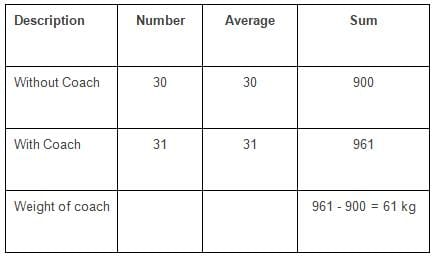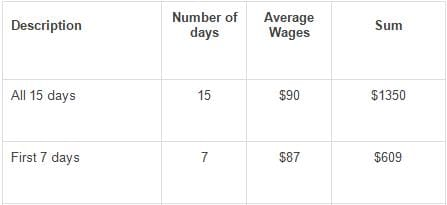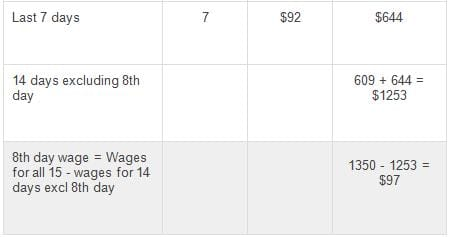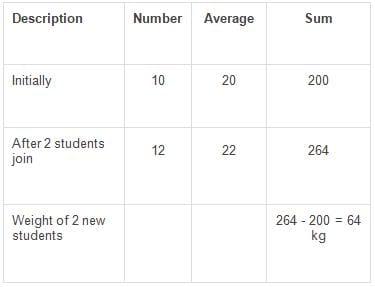# Test: Statistics- 3

## 10 Questions MCQ Test Quantitative Aptitude for GMAT | Test: Statistics- 3

Description
Attempt Test: Statistics- 3 | 10 questions in 20 minutes | Mock test for GMAT preparation | Free important questions MCQ to study Quantitative Aptitude for GMAT for GMAT Exam | Download free PDF with solutions
QUESTION: 1

### If the mean of numbers 28, x, 42, 78 and 104 is 62, what is the mean of 48, 62, 98, 124 and x?

Solution:

x is common to both the series. So, x is not going to make a difference to the average.

Only the remaining 4 numbers will contribute to the difference in average between the two series.

Sum of the 4 numbers, excluding x, of the first series is 28 + 42 + 78 + 104 = 252

Sum of the 4 numbers, excluding x, of the second series is 48 + 62 + 98 + 124 = 332

The difference between the sum of the two sets of numbers = 332 - 252 = 80

The sum of the second series is 80 more than the sum of the first series.

If the sum of the second series is 80 more, the average of the second series will be 80/5 = 16 more than the first series.

Therefore, the average of the second series = 62 + 16 = 78.

QUESTION: 2

### The arithmetic mean of the 5 consecutive integers starting with 's' is 'a'. What is the arithmetic mean of 9 consecutive integers that start with s + 2?

Solution:

The sequence starts with 's' and its mean is 'a'

The mean of 5 consecutive numbers is the 3rd term - the middle term.

Hence, 'a' the mean is the middle (3rd) term.

The terms of the sequence are s, s + 1, s + 2, s + 3, and s + 4

The middle term is s + 2

Therefore, a = s + 2

The second series starts from s + 2

The terms will therefore, be s + 2, s + 3, s + 4, s + 5, s + 6, s + 7, s + 8, s + 9, and s + 10

The middle term of the second series is the 5th term = s + 6

If a = s + 2, then s + 6 will be a + 4

The average of the second sequence is a + 4

QUESTION: 3

### The average weight of a group of 30 friends increases by 1 kg when the weight of their football coach was added. If average weight of the group after including the weight of the football coach is 31 kg, what is the weight of their football coach?

Solution:

The new average weight of the group after including the football coach = 31 kg.
As the new average is 1 kg more than the old average, old average without including the football coach = 30 kg.The total weight of the 30 friends without including the football coach = 30 * 30 = 900.
After including the football coach, the number people in the group increases to 31 and the average weight of the group increases by 1 kg.

Therefore, the total weight of the group after including the weight of the football coach = 31 * 31 = 961 kg.
Therefore, the weight of the football coach = 961 - 900 = 61 kg.

Choice B is the correct answer.

QUESTION: 4

The average wages of a worker during a fortnight comprising 15 consecutive working days was \$90 per day. During the first 7 days, his average wages was \$87/day and the average wages during the last 7 days was \$92 /day. What was his wage on the 8th day?

Solution:

Fill in the data given in the question in the standard framework as shown below. Most questions in averages can be solved by completing this standard framework. The sum of wages is computed as the product of the number of days and the average wage earned per day.The total wages earned during the 15 days that the worker worked = 15 * 90 = \$ 1350.

The total wages earned during the first 7 days = 7 * 87 = \$ 609.
The total wages earned during the last 7 days = 7 * 92 = \$ 644.

Total wages earned during the 15 days = wages during first 7 days + wage on 8th day + wages during the last 7 days.
Or 1350 = 609 + wage on 8th day + 644
Wage on 8th day = 1350 - 609 - 644 = \$ 97.

Choice D is the correct answer.

QUESTION: 5

The average of 5 quantities is 6. The average of 3 of them is 8. What is the average of the remaining two numbers?

Solution:

The average of 5 quantities is 6.
Therefore, the sum of the 5 quantities is 5 * 6 = 30.
The average of three of these 5 quantities is 8.
Therefore, the sum of these three quantities = 3 * 8 = 24
The sum of the remaining two quantities = (Sum of all 5 - Sum of 3 quantities) = 30 - 24 = 6.
Average of these two quantities = 6/2 = 3
Choice C is the correct answer.

QUESTION: 6

The average age of a group of 10 students was 20. The average age increased by 2 years when two new students joined the group. What is the average age of the two new students who joined the group?

Solution:The average age of a group of 10 students is 20.
Therefore, the sum of the ages of all 10 of them = 10 * 20 = 200

When two new students join the group, the average age increases by 2. New average = 22.
Now, there are 12 students.
Therefore, the sum of the ages of all 12 of them = 12 * 22 = 264

Therefore, the sum of the ages of the two new students who joined = 264 - 200 = 64

And the average age of each of the two new students = 64/2 = 32 years

Choice D is the correct answer.

QUESTION: 7

Positive integers from 1 to 45, inclusive are placed in 5 groups of 9 each. What is the highest possible average of the medians of these 5 groups?

Solution:

We need to maximize the median in each group in order to maximize the average of all the medians.

The highest possible median is 41 as there should be 4 numbers higher than the median in a group of 9.
So, if we have a group that has a, b, c, d, 41, 42, 43, 44, 45, the median will be 41.
In this set, it is essential not to expend any more high values on a, b, c, or d as these do not affect the median.

The median of a group that comprises 1, 2, 3, 4, 41, 42, 43, 44, 45 will be 41.

The next group can be 5, 6, 7, 8, 36, 37, 38, 39, 40. The median will be 36.

Extrapolating the findings in the two sets listed above, to maximize medians in all the 5 groups, the medians of the 5 groups will have to be 21, 26, 31, 36 and 41.

The average of the highest possible medians will be the average of these 5 numbers = 31.

Choice B is the correct answer.

QUESTION: 8

If the average of 5 positive integers is 40 and the difference between the largest and the smallest of these 5 numbers is 10, what is the maximum value possible for the largest of these 5 integers?

Solution:

The average of 5 positive integers is 40. i.e., the sum of these integers = 5 * 40 = 200

Let the least of these 5 numbers be x.

Because the range of the set is 10, the largest of these 5 numbers will be x + 10.

If we have to maximize the largest of these numbers, we have to minimize all the other numbers.

That is 4 of these numbers are all at the least value possible = x.

So, x + x + x + x + x + 10 = 200

Or x = 38.

So, the maximum value possible for the largest of these 5 integers is 48.

Choice D is the correct answer.

QUESTION: 9

An analysis of the monthly incentives received by 5 salesmen : The mean and median of the incentives is \$7000. The only mode among the observations is \$12,000. Incentives paid to each salesman were in full thousands. What is the difference between the highest and the lowest incentive received by the 5 salesmen in the month?

Solution:

The arithmetic mean of the incentives is \$7000.
The median of the incentives is also \$7000.
There is only one mode and the mode is \$12,000.
Let their incentives be a, b, c, d, and e such that a ≤ b ≤ c ≤ d ≤ e

Therefore, the median of these values is 'c'.

So, c = \$7000.

The sum of their incentives a + b + c + d + e = 5 * 7000 = \$35,000

There is only one mode amongst these 5 observations.

The mode is that value that appears with the maximum frequency.

Hence, \$12,000 is the incentive received by the most number of salesmen.

The incentive that c has got is \$7000

So, the incentive received by d and e has to be greater than or equal to \$7000

But the mode is \$12,000

So, d and e should have got \$12,000 each.

Therefore, c + d + e = 7000 + 12,000 + 12,000 = \$31,000

Hence, a + b = 35,000 - 31,000 = \$4000

As there is only one mode, the incentives received by a and b have to be different

Maximum incentive : \$12,000

Minimum incentive : \$1000

Difference between maximum and minimum incentive : \$11,000

QUESTION: 10

Data Sufficiency:
If m, s are the average and standard deviation of integers a, b, c, and d, is s > 0?

1. m > a
2. a + b + c + d = 0
Solution:

Statement 1: m > a

If a = b = c = d, the average m will be the same as a.

Since m > a, all the elements in the set cannot be the same, and therefore, s > 0.

Statement 1 ALONE is sufficient.

Eliminate choices B, C and E. Choices narrow down to A and D.Use Code STAYHOME200 and get INR 200 additional OFF Use Coupon Code